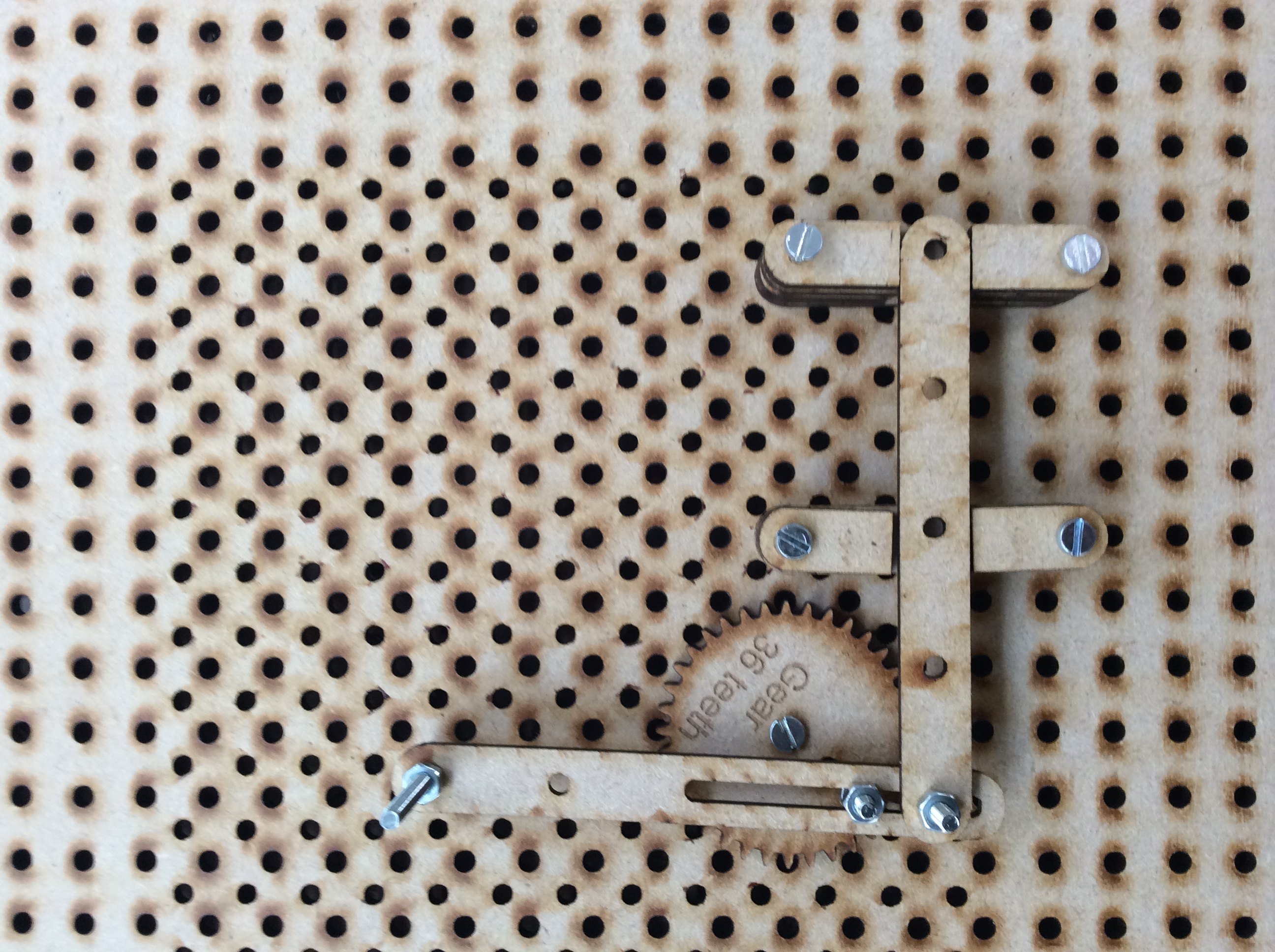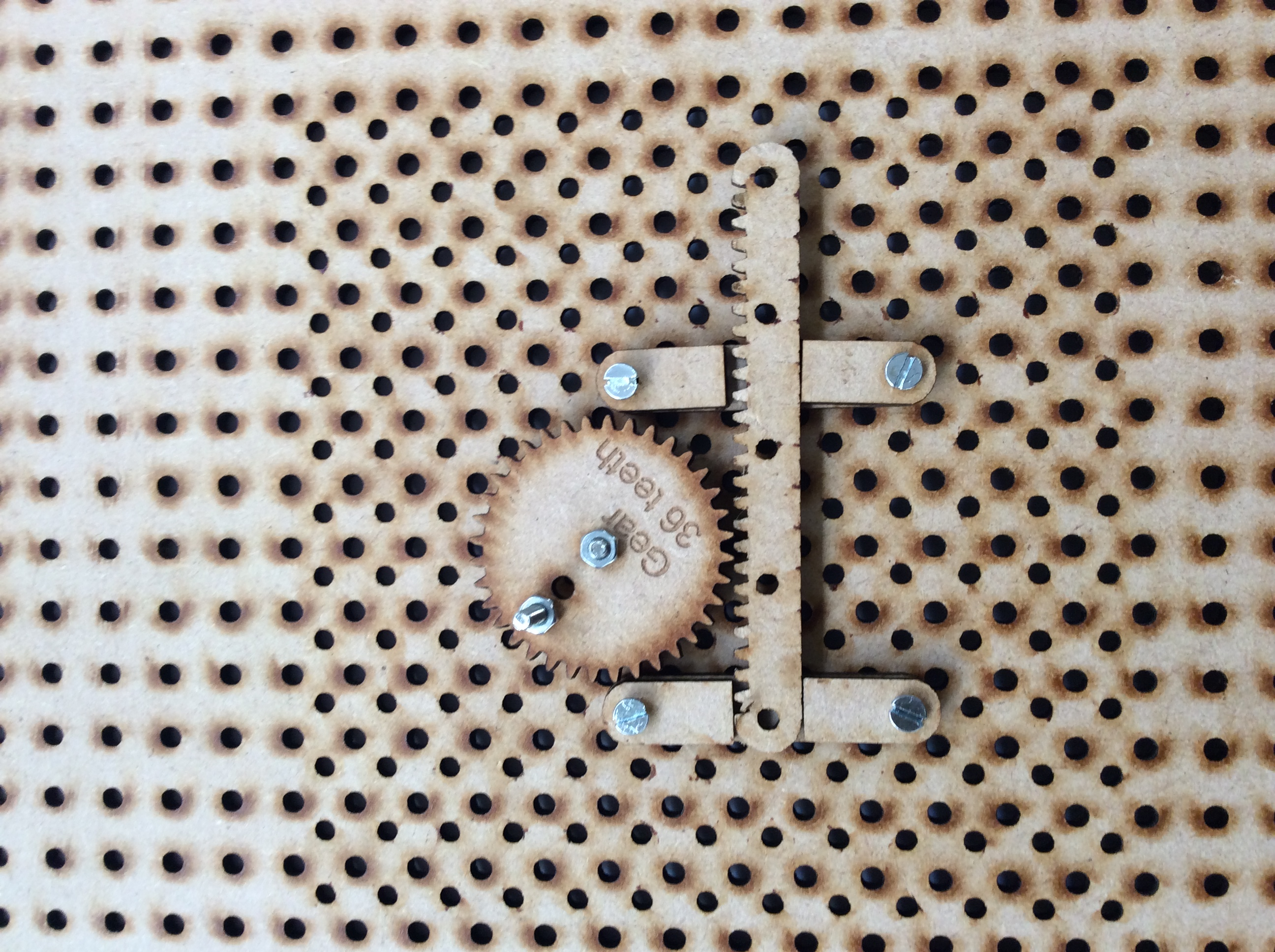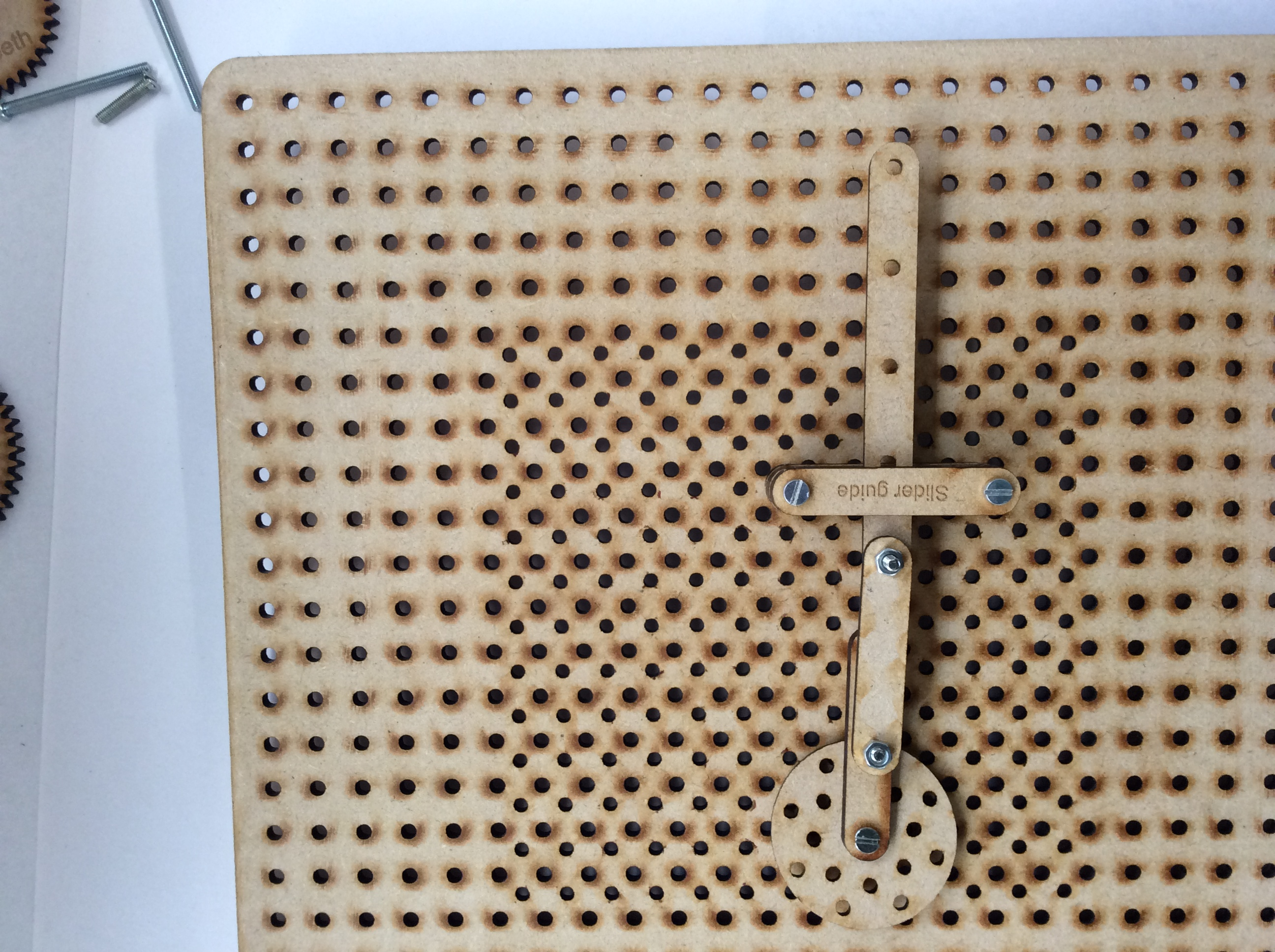# An Introduction to Mechanisms

### Learn It

• Linkages are mechanisms which allow engineers to alter force and/or motion to perform useful functions.
• We can use linkages to change:
• The direction of motion
• The type of motion
• The size of a force
• The motion and/or force that is input at one end of a mechanical linkages is different from the output.

### Learn It - Reverse-motion linkage

• A reverse-motion linkage changes the direction of motion.
• The reverse-motion linkage in the video below, alters the direction of motion. Pushing as input, becomes pulling as output, and vice versa

1. Replicate the reverse-motion linkage shown above using Wacano
2. Adjust the pivot, so that a small input displacement becomes a large output displacement.
3. Adjust the pivot, so that a small input force becomes a large output force. Take a photo of this one and upload your work.

### Learn It - Parallel-motion linkage

• A parallel-motion linkage creates an identical parallel motion with regard to direction.

• The setup above shows a parallel linkage that transfers identical motion.
• By adjusting the pivot points, we can alter sizes of motion and/or forces.

• Set up a parallel linkage using Wacono, to give provide the largest possible force on the rubber band.
• If an input force of 5N moving a distance of 10cm was used, what would be the output force if the output distance was 2cm?

### Learn It - Bell-crank linkage

• A bell-crank linkage changes the direction of movement through 90°.
• A bell-crank linkage looks a like an "L".
• By pulling (or pushing) the linkage in one direction, it creates a similar motion at the other end of the linkage at 90°.

• A treadle linkage shows how linkages can be used to change one type of motion into another.
• In this case, the rotary motion of the cam moves a parallel-motion linkage.
• The parallel-motion linkage controls the identical side-to-side, or oscillating motion.